# Resources tagged with: GeoGebra

Filter by: Content type:
Age range:
Challenge level:

There are 81 NRICH Mathematical resources connected to GeoGebra, you may find related items under Physical and Digital Manipulatives.

Broad Topics > Physical and Digital Manipulatives > GeoGebra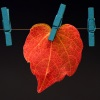### Number Lines in Disguise

##### Age 7 to 14 Challenge Level:

Some of the numbers have fallen off Becky's number line. Can you figure out what they were?### Robotic Rotations

##### Age 11 to 16 Challenge Level:

How did the the rotation robot make these patterns?### Polygon Rings

##### Age 11 to 14 Challenge Level:

Join pentagons together edge to edge. Will they form a ring?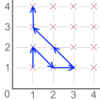### Areas from Vectors

##### Age 11 to 16

Use the applet to explore the area of a parallelogram and how it relates to vectors.### Coordinates of Corners

##### Age 11 to 16

Use the applet to make some squares. What patterns do you notice in the coordinates?### Diminishing Returns

##### Age 11 to 14 Challenge Level:

How much of the square is coloured blue? How will the pattern continue?##### Age 11 to 16 Challenge Level:

Draw some quadrilaterals on a 9-point circle and work out the angles. Is there a theorem?### Coded Hundred Square

##### Age 7 to 11 Challenge Level:

This 100 square jigsaw is written in code. It starts with 1 and ends with 100. Can you build it up?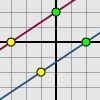### Translating Lines

##### Age 11 to 14 Challenge Level:

Investigate what happens to the equation of different lines when you translate them. Try to predict what will happen. Explain your findings.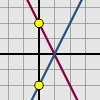### Reflecting Lines

##### Age 11 to 14 Challenge Level:

Investigate what happens to the equations of different lines when you reflect them in one of the axes. Try to predict what will happen. Explain your findings.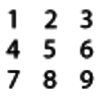### Four-digit Targets

##### Age 7 to 11 Challenge Level:

You have two sets of the digits 0 – 9. Can you arrange these in the five boxes to make four-digit numbers as close to the target numbers as possible?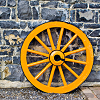### Interactive Spinners

##### Age 11 to 14 Challenge Level:

This interactivity invites you to make conjectures and explore probabilities of outcomes related to two independent events.### More Twisting and Turning

##### Age 11 to 16 Challenge Level:

It would be nice to have a strategy for disentangling any tangled ropes...### Round and Round and Round

##### Age 11 to 14 Challenge Level:

Where will the point stop after it has turned through 30 000 degrees? I took out my calculator and typed 30 000 ÷ 360. How did this help?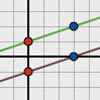### Parallel Lines

##### Age 11 to 14 Challenge Level:

How does the position of the line affect the equation of the line? What can you say about the equations of parallel lines?### Multiplication Square Jigsaw

##### Age 7 to 11 Challenge Level:

Can you complete this jigsaw of the multiplication square?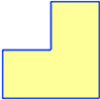### Twice as Big?

##### Age 7 to 11 Challenge Level:

Investigate how the four L-shapes fit together to make an enlarged L-shape. You could explore this idea with other shapes too.### More Magic Potting Sheds

##### Age 11 to 14 Challenge Level:

The number of plants in Mr McGregor's magic potting shed increases overnight. He'd like to put the same number of plants in each of his gardens, planting one garden each day. How can he do it?### Magic Potting Sheds

##### Age 11 to 14 Challenge Level:

Mr McGregor has a magic potting shed. Overnight, the number of plants in it doubles. He'd like to put the same number of plants in each of three gardens, planting one garden each day. Can he do it?### More Transformations on a Pegboard

##### Age 7 to 11 Challenge Level:

Use the interactivity to find all the different right-angled triangles you can make by just moving one corner of the starting triangle.### Semi-regular Tessellations

##### Age 11 to 16 Challenge Level:

Semi-regular tessellations combine two or more different regular polygons to fill the plane. Can you find all the semi-regular tessellations?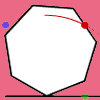### Up and Across

##### Age 11 to 14 Challenge Level:

Experiment with the interactivity of "rolling" regular polygons, and explore how the different positions of the dot affects its vertical and horizontal movement at each stage.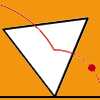### Speeding Up, Slowing Down

##### Age 11 to 14 Challenge Level:

Experiment with the interactivity of "rolling" regular polygons, and explore how the different positions of the red dot affects its speed at each stage.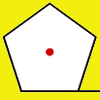### How Far Does it Move?

##### Age 11 to 14 Challenge Level:

Experiment with the interactivity of "rolling" regular polygons, and explore how the different positions of the red dot affects the distance it travels at each stage.### Mixing Paints

##### Age 11 to 14 Challenge Level:

Can you work out how to produce different shades of pink paint?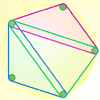### Board Block Challenge

##### Age 7 to 11 Challenge Level:

Choose the size of your pegboard and the shapes you can make. Can you work out the strategies needed to block your opponent?### Nine-pin Triangles

##### Age 7 to 11 Challenge Level:

How many different triangles can you make on a circular pegboard that has nine pegs?### Right Angles

##### Age 11 to 14 Challenge Level:

Can you make a right-angled triangle on this peg-board by joining up three points round the edge?### Subtended Angles

##### Age 11 to 14 Challenge Level:

What is the relationship between the angle at the centre and the angles at the circumference, for angles which stand on the same arc? Can you prove it?### Triangles in Circles

##### Age 11 to 14 Challenge Level:

Can you find triangles on a 9-point circle? Can you work out their angles?### Partitioning Revisited

##### Age 11 to 14 Challenge Level:

We can show that (x + 1)² = x² + 2x + 1 by considering the area of an (x + 1) by (x + 1) square. Show in a similar way that (x + 2)² = x² + 4x + 4### Square Coordinates

##### Age 11 to 14 Challenge Level:

A tilted square is a square with no horizontal sides. Can you devise a general instruction for the construction of a square when you are given just one of its sides?### Isosceles Triangles

##### Age 11 to 14 Challenge Level:

Draw some isosceles triangles with an area of $9$cm$^2$ and a vertex at (20,20). If all the vertices must have whole number coordinates, how many is it possible to draw?### Picturing Triangular Numbers

##### Age 11 to 14 Challenge Level:

Triangular numbers can be represented by a triangular array of squares. What do you notice about the sum of identical triangle numbers?### Rollin' Rollin' Rollin'

##### Age 11 to 14 Challenge Level:

Two circles of equal radius touch at P. One circle is fixed whilst the other moves, rolling without slipping, all the way round. How many times does the moving coin revolve before returning to P?### Rolling Around

##### Age 11 to 14 Challenge Level:

A circle rolls around the outside edge of a square so that its circumference always touches the edge of the square. Can you describe the locus of the centre of the circle?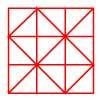### Fractional Triangles

##### Age 7 to 11 Challenge Level:

Use the lines on this figure to show how the square can be divided into 2 halves, 3 thirds, 6 sixths and 9 ninths.### Farey Sequences

##### Age 11 to 14 Challenge Level:

There are lots of ideas to explore in these sequences of ordered fractions.### A Square of Numbers

##### Age 7 to 11 Challenge Level:

Can you put the numbers 1 to 8 into the circles so that the four calculations are correct?### Reflecting Squarely

##### Age 11 to 14 Challenge Level:

In how many ways can you fit all three pieces together to make shapes with line symmetry?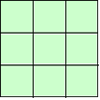### 1, 2, 3 Magic Square

##### Age 7 to 11 Challenge Level:

Arrange three 1s, three 2s and three 3s in this square so that every row, column and diagonal adds to the same total.### Transformations on a Pegboard

##### Age 7 to 11 Challenge Level:

How would you move the bands on the pegboard to alter these shapes?### Estimating Angles

##### Age 7 to 14 Challenge Level:

How good are you at estimating angles?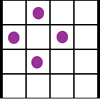### Square Corners

##### Age 7 to 11 Challenge Level:

What is the greatest number of counters you can place on the grid below without four of them lying at the corners of a square?### Factor Lines

##### Age 7 to 14 Challenge Level:

Arrange the four number cards on the grid, according to the rules, to make a diagonal, vertical or horizontal line.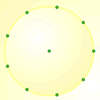##### Age 7 to 11 Challenge Level:

How many DIFFERENT quadrilaterals can be made by joining the dots on the 8-point circle?### Which Scripts?

##### Age 7 to 11 Challenge Level:

There are six numbers written in five different scripts. Can you sort out which is which?### Nine Colours

##### Age 11 to 16 Challenge Level:

Can you use small coloured cubes to make a 3 by 3 by 3 cube so that each face of the bigger cube contains one of each colour?### Is There a Theorem?

##### Age 11 to 14 Challenge Level:

Draw a square. A second square of the same size slides around the first always maintaining contact and keeping the same orientation. How far does the dot travel?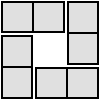### 4 Dom

##### Age 5 to 16 Challenge Level:

Use these four dominoes to make a square that has the same number of dots on each side.